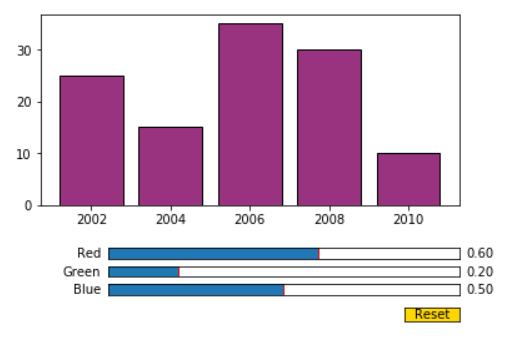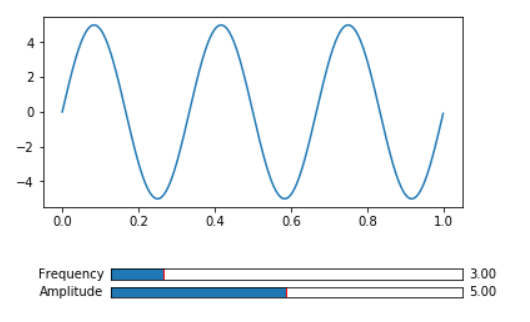Related Articles
Matplotlib – Slider Widget
• Last Updated : 23 Feb, 2021

Matplotlib provides several widgets to make interactive plots. Among these widgets, the Slider widget is discussed here. The Slider provides control over the visual properties of the plot.  Slider() is used to place a slider representing a floating point range in a plot on provided axes.

Syntax:

class matplotlib.widgets.Slider(ax, label, valmin, valmax, valinit=0.5, valfmt=None, closedmin=True, closedmax=True, slidermin=None, slidermax=None, dragging=True, valstep=None, orientation=’horizontal’, **kwargs)

Parameters:

1. ax: A matplotlib.axes.Axes instance where a slider is placed
2. label: Slider text label
3. valmin: The minimum value of the slider
4. valmax: The maximum value of the slider
5. valinit: Initial Value of a slider. Default value is 0.5.
6. valfmt: Slider value format string (%-format). Default value is None. If None, a ScalarFormatter is used.
7. closedmin: Slider interval is closed on bottom or not.
8. closedmax: Slider interval is closed on the top or not.
9. slidermin: Forbid current slider to have value less than current value of the given slider. Default value is None.
10. slidermax: Forbid current slider to have value greater than current value of the given slider. Default value is None.
11. dragging: The slider can be dragged by mouse or not. Default value is True (slider can be dragged by mouse)
12. valstep: The slider will slides at values in multiples of valstep value. Default value is None.
13. orientation: The slider orientation, vertical or horizontal . Default value is horizontal.
14. kwargs are related to Rectangle that draws the slider knob. Valid properties such as facecolor, edgecolor, alpha, etc. of Matplotlib.patches.Rectangle can be used here.

Methods:

• disconnect(self, cid): Removes the observer with connection id cid
• on_changed(self, func): To connect to the slider event. When the slider value is changed, respective function func is called. Func takes a new slider value as argument and returns connection id.
• reset(self):  The slider value is set to the initial value
• set_val(self, val): Sets slider value to val

Example 1:

Following example demonstrates the change in color of bar chart using reg, green, blue value sliders.

## Python3

 `# Import libraries``import` `numpy as np``import` `matplotlib.pyplot as plt``from` `matplotlib.widgets ``import` `Slider, Button`` ` `# Create a subplot``fig, ax ``=` `plt.subplots()``plt.subplots_adjust(bottom``=``0.35``)``r ``=` `0.6``g ``=` `0.2``b ``=` `0.5`` ` `# Create and plot a bar chart``year ``=` `[``'2002'``, ``'2004'``, ``'2006'``, ``'2008'``, ``'2010'``]``production ``=` `[``25``, ``15``, ``35``, ``30``, ``10``]``plt.bar(year, production, color``=``(r, g, b),``        ``edgecolor``=``"black"``)`` ` `# Create 3 axes for 3 sliders red,green and blue``axred ``=` `plt.axes([``0.25``, ``0.2``, ``0.65``, ``0.03``])``axgreen ``=` `plt.axes([``0.25``, ``0.15``, ``0.65``, ``0.03``])``axblue ``=` `plt.axes([``0.25``, ``0.1``, ``0.65``, ``0.03``])`` ` `# Create a slider from 0.0 to 1.0 in axes axred``# with 0.6 as initial value.``red ``=` `Slider(axred, ``'Red'``, ``0.0``, ``1.0``, ``0.6``)`` ` `# Create a slider from 0.0 to 1.0 in axes axgreen``# with 0.2 as initial value.``green ``=` `Slider(axgreen, ``'Green'``, ``0.0``, ``1.0``, ``0.2``)`` ` `# Create a slider from 0.0 to 1.0 in axes axblue``# with 0.5(default) as initial value``blue ``=` `Slider(axblue, ``'Blue'``, ``0.0``, ``1.0``)`` ` `# Create fuction to be called when slider value is changed`` ` `def` `update(val):``    ``r ``=` `red.val``    ``g ``=` `green.val``    ``b ``=` `blue.val``    ``ax.bar(year, production, color``=``(r, g, b),``           ``edgecolor``=``"black"``)`` ` `# Call update function when slider value is changed``red.on_changed(update)``green.on_changed(update)``blue.on_changed(update)`` ` `# Create axes for reset button and create button``resetax ``=` `plt.axes([``0.8``, ``0.025``, ``0.1``, ``0.04``])``button ``=` `Button(resetax, ``'Reset'``, color``=``'gold'``,``                ``hovercolor``=``'skyblue'``)`` ` `# Create a fucntion resetSlider to set slider to``# initial values when Reset button is clicked`` ` `def` `resetSlider(event):``    ``red.reset()``    ``green.reset()``    ``blue.reset()`` ` `# Call resetSlider function when clicked on reset button``button.on_clicked(resetSlider)`` ` `# Display graph``plt.show()`

Output:Example 2:

In this example, a slider are used to change the frequency and amplitude of a sine wave

## Python3

 `# Import libraries``import` `numpy as np``import` `matplotlib.pyplot as plt``from` `matplotlib.widgets ``import` `Slider, Button`` ` `# Create subplot``fig, ax ``=` `plt.subplots()``plt.subplots_adjust(bottom``=``0.35``)`` ` `# Create and plot sine wave``t ``=` `np.arange(``0.0``, ``1.0``, ``0.001``)``s ``=` `5` `*` `np.sin(``2` `*` `np.pi ``*` `3` `*` `t)``l, ``=` `plt.plot(t, s)`` ` `# Create axes for frequency and amplitude sliders``axfreq ``=` `plt.axes([``0.25``, ``0.15``, ``0.65``, ``0.03``])``axamplitude ``=` `plt.axes([``0.25``, ``0.1``, ``0.65``, ``0.03``])`` ` `# Create a slider from 0.0 to 20.0 in axes axfreq``# with 3 as initial value``freq ``=` `Slider(axfreq, ``'Frequency'``, ``0.0``, ``20.0``, ``3``)`` ` `# Create a slider from 0.0 to 10.0 in axes axfreq``# with 5 as initial value and valsteps of 1.0``amplitude ``=` `Slider(axamplitude, ``'Amplitude'``, ``0.0``,``                   ``10.0``, ``5``, valstep``=``1.0``)`` ` `# Create fuction to be called when slider value is changed`` ` `def` `update(val):``    ``f ``=` `freq.val``    ``a ``=` `amplitude.val``    ``l.set_ydata(a``*``np.sin(``2``*``np.pi``*``f``*``t))`` ` `# Call update function when slider value is changed``freq.on_changed(update)``amplitude.on_changed(update)`` ` `# display graph``plt.show()`

Output:Attention geek! Strengthen your foundations with the Python Programming Foundation Course and learn the basics.

To begin with, your interview preparations Enhance your Data Structures concepts with the Python DS Course.

My Personal Notes arrow_drop_up